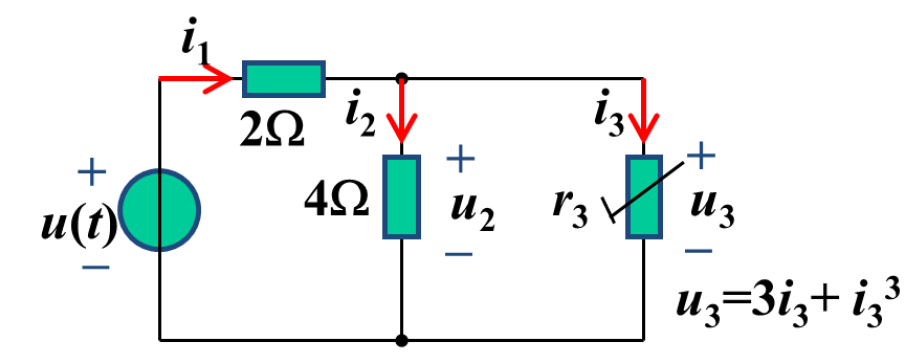【简答题】For the circuit, we knowu(t)=8V+4.4sinωtmV. The working point ofr3is(__V,__A) and the small signalΔu3=__sinωtmV.A.

B.

C.

D.

E.

A.

B.

C.

D.

A.

B.

C.

D.

E.

A.

B.

C.

D.

A.

B.

C.

D.

E.

A.

B.

C.

D.

E.

( )应当将施工图设计文件报建设行政主管部门审查，施工图设计文件未经审查批准的，不得使用。
A.

B.

C.

D.

[多选题]关于价值工程基本原理的说法，正确的有()
A.

B.

C.

D.

E.

( )反映融资成本的基本要素，对于贷款是利息，对于租赁是租金，对于债券是债息。
A.

B.

C.

D.

A.

B.

C.

D.

A.

B.

C.

D.

E.

A.

B.

C.

D.

A.

B.

C.

D.

[单选题]某固定资产原价为60000元，预计净残值为800元，预计使用年限5年，若采用双倍额递减法计提折旧，则第2年的折旧额为()元。
A.
14400
B.
19400
C.
20800
D.
24000
( )是项目公司资本结构决策的依据。
A.

B.

C.

D.

A.

B.

C.

D.

A.
1
B.
2
C.
3
D.
4

A.

B.

C.

D.

A.
2
B.
3
C.
4
D.
5

A.

B.

C.

D.

[单选题]根据《招标投标法实施条例》，属于招标人与投标人串标的是()
A.

B.

C.

D.

A.
5
B.
4
C.
3
D.
2

A.
TOT
B.
ABS
C.
BOT
D.
PPP

A.

B.

C.

D.

E.

BOT融资方式需要政府参与是(　　)。
A.

B.

C.

D.

E.

A.

B.

C.

D.

A.

B.

C.

D.

E.

A.

B.

C.

D.

A.
2050
B.
2170
C.
2230
D.
2290

A.

B.

C.

D.

[单选题]关于勘察、设计单位的质量责任和义务的说法，错误的是()
A.

B.

C.

D.

A.
14
B.
15
C.
16
D.
17

A.

B.

C.

D.

E.

A.

B.

C.

D.

A.
14
B.
28
C.
42
D.
56

A.

B.

C.

D.

E.

A.
45%
B.
50%
C.
55%
D.
60%

A.

B.

C.

D.

[单选题]进行工程项目财务分析时，营业收入应包括()
A.

B.

C.

D.

A.

B.

C.

D.

A.
①——②——③
B.
②——①——③
C.
②——③——①
D.
③——②——①
[多选题]采用定率估算法编制成本计划时，确定子项目成本降低率可采用的方法有()
A.

B.

C.

D.

E.

A.
7
B.
21
C.
14
D.
28

A.
7
B.
14
C.
28
D.
40
( )是从BOT方式演变而来的一种新型方式，具体是指用民营资金购买某个项目资金的经营权，购买者在约定的时间内通过经营该资产收回全部投资和得到合理的回报后再将项目无偿移交给原产权所有人。
A.
TOT
B.
ABS
C.
PPP
D.
BOT

A.

B.

C.

D.

A.

B.

C.

D.

A.

B.

C.

D.

A.

B.

C.

D.

[单选题]单位工程施工组织设计应由()主持编制。
A.

B.

C.

D.

A.

B.

C.

D.

E.

A.

B.

C.

D.

[单选题]下列工程项目中，可以不进行经济费用效益分析的是()
A.

B.

C.

D.

A.

B.

C.

D.

A.

B.

C.

D.

A.
0.136
B.
0.179
C.
0.224
D.
0.298

A.

B.

C.

D.

A.
30万元
B.
33.66万元
C.
29.4万元
D.
25.5万元

A.

B.

C.

D.

[多选题]下列选项中，属于效力待定合同的有()
A.

B.

C.

D.

E.

A.

B.

C.

D.

[单选题]具有撤销权的当事人自知道或者应当知道撤销事由之日起()年内没有行使撤销权，该撤销权消灭。
A.
1
B.
2
C.
3
D.
5

A.

B.

C.

D.

A.

B.

C.

D.

A.

B.

C.

D.

E.
ABC分析法

A.

B.

C.

D.

A.

B.

C.

D.

A.

B.

C.

D.

A.

B.

C.

D.

A.

B.

C.

D.

A、B工作完成后C工作开始，C工作完成后D、E两项工作开始。已知A、B、C、D、E五项工作持续时间分别为3、2、4，3、5天，A、B工作最早开始时间分别为8、12天，D、E工作最迟完成时间分别为25、26天，则A工作的最迟开始时间应为（　　）。
A.
11天
B.
14天
C.
19天
D.
17天

A.

B.

C.
CM单位与分包商以简单的成本加酬金
D.
CM单位与分包商以保证最大工程费用加酬金

A.

B.

C.

D.

[单选题]影响利率的因素有多种，通常情况下，利率的最高界线是()
A.

B.

C.

D.

A.

B.

C.

D.

A.

B.

C.

D.

A.

B.

C.

D.

A.

B.

C.

D.

A.

B.

C.

D.

A.

B.

C.

D.

E.

A.

B.

C.

D.

E.

A.

B.
A工作总时差为5天
C.
A工作自由时差为2天
D.
B工作总时差为2天
E.
B工作自由时差为1天
[单选题]某工程项目由四栋房屋组成，在进行流水作业施工时，4个施工过程的流水节拍分别为8周、4周、8周、8周，则流水步距为()周。
A.
1
B.
2
C.
4
D.
8

A.

B.

C.

D.

A.

B.

C.

D.
8h内下沉量小于或等于10mm时，可以封底

A.
100m
B.
100m3
C.
10m3
D.
m3

A.

B.

C.

D.

E.

A.

B.

C.

D.

E.

A.
60%
B.
70%
C.
80%
D.
90%

A.

B.

C.

D.

A.
1
B.
2
C.
3
D.
4

A.

B.

C.

D.

A.

B.

C.

D.

E.

A.
14
B.
28
C.
42
D.
56

A.

B.

C.

D.

E.

《建设工程工程量清单计价规范》，关于项目特征，说法正确的是(　)。
A.

B.

C.

D.

A.

B.

C.

D.

A.

B.

C.

D.

A.

B.

C.

D.

A.

B.

C.

D.打开"刷刷题APP"，使用更方便